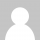# Hacker News 排名算法工作原理

### 深入 news.arc 程序代码

Hacker News是用Arc语言开发的，这是一种Lisp方言，由Y Combinator投资公司创始人Paul Graham创造。Hacker News的开源的，你可以在arclanguage.org找到它的源代码。深入发掘 news.arc 程序，你会找到这段排名算法代码，就是下面这段：

```; Votes divided by the age in hours to the gravityth power.
; Would be interesting to scale gravity in a slider.

(= gravity* 1.8 timebase* 120 front-threshold* 1
nourl-factor* .4 lightweight-factor* .3 )

(def frontpage-rank (s (o scorefn realscore) (o gravity gravity*))
(* (/ (let base (- (scorefn s) 1)
(if (> base 0) (expt base .8) base))
(expt (/ (+ (item-age s) timebase*) 60) gravity))
(if (no (in s!type 'story 'poll))  1
(blank s!url)                  nourl-factor*
(lightweight s)                (min lightweight-factor*
(contro-factor s))
(contro-factor s))))```

```Score = (P-1) / (T+2)^G

P = 文章获得的票数( -1 是去掉文章提交人的票)
T = 从文章提交至今的时间(小时)
G = 比重，news.arc里缺省值是1.8```

### 比重(G)和时间(T)对排名的影响

• 当T增加时文章得分会下降，这就是说越老的文章分数会越底。
• 当比重加大时，老的文章的得分会减的更快

### 得分随着时间是如何变化的```plot(
(30 - 1) / (t + 2)^1.8,
(60 - 1) / (t + 2)^1.8,
(200 - 1) / (t + 2)^1.8
) where t=0..24```

### 比重参数是如何影响排名的

```plot(
(p - 1) / (t + 2)^1.8,
(p - 1) / (t + 2)^0.5,
(p - 1) / (t + 2)^2.0
) where t=0..24, p=10```

### Python语言实现

```def calculate_score(votes, item_hour_age, gravity=1.8):
return (votes - 1) / pow((item_hour_age+2), gravity)```

Paul Graham 分享了修正后的HN 排名算法

```    (= gravity* 1.8 timebase* 120 front-threshold* 1
nourl-factor* .4 lightweight-factor* .17 gag-factor* .1)

(def frontpage-rank (s (o scorefn realscore) (o gravity gravity*))
(* (/ (let base (- (scorefn s) 1)
(if (> base 0) (expt base .8) base))
(expt (/ (+ (item-age s) timebase*) 60) gravity))
(if (no (in s!type 'story 'poll))  .8
(blank s!url)                  nourl-factor*
(mem 'bury s!keys)             .001
(* (contro-factor s)
(if (mem 'gag s!keys)
gag-factor*
(lightweight s)
lightweight-factor*
1)))))```

### 4 Responses to Hacker News 排名算法工作原理

1.超神肉肉 says:

看起来挺酷的呀！！！！

2.jacky says:

为什么不贴出修正后的算法的py的代码呢？

3.geek42 says:

关键在于他的计算触发策略是怎样

是 每次浏览请求时候把所有的算一遍？
还是 每隔一定时间把所有的算一遍？

4. pharmacist&programmer 对这篇文章的反应是飘过~